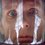# Playing with Integrals: Limits and Integrals 1

As I said in previous post the formal definition of the Riemann Integral is very useful when solving Olympiad problems.

Problem 1. Find the following limit $\lim_{n\to\infty}\left(\frac{1}{n+1}+\frac{1}{n+2}+...+\frac{1}{2n}\right)$

Solution. Let try to make our sum into something more or less similar to Riemann sum. $\sum_{i=1}^n\frac{1}{n+i}=\frac{1}{n}\left(\sum_{i=1}^n\frac{1}{1+\frac{i}{n}}\right).$

Does this remind you of the monstrous $\displaystyle\sum_{i=1}^n f(\xi_i)(x_i-x_{i-1})$? But what if set $x_i=\dfrac{i}{n}$ and consider the right Riemann sum? Now it will transform into $\dfrac{1}{n}\left(\displaystyle\sum_{i=1}^n f\left(\dfrac{i}{n}\right)\right)$.

From the last formula we can easily understand what function $f$ we need to consider, so by the definition: $\lim_{n\to\infty}\left(\frac{1}{n+1}+\frac{1}{n+2}+...+\frac{1}{2n}\right)=\frac{1}{n}\left(\sum_{i=1}^n\frac{1}{1+\frac{i}{n}}\right)=$ $=\dfrac{1}{n}\left(\displaystyle\sum_{i=1}^n f\left(\dfrac{i}{n}\right)\right)=\int^1_0\frac{1}{x+1}=\boxed{\ln 2}.$

Now using the same approach try solving the following problems.

Problem 2. $\lim_{n\to\infty}\left(\frac{1}{n+1}+\frac{1}{n+2}+...+\frac{1}{4n}\right)$

Problem 3. $\lim_{n\to\infty}\left(\frac{n}{n^2+1}+\frac{n}{n^2+4}+...+\frac{n}{n^2+n^2}\right)$Note by Nicolae Sapoval
6 years, 2 months ago

This discussion board is a place to discuss our Daily Challenges and the math and science related to those challenges. Explanations are more than just a solution — they should explain the steps and thinking strategies that you used to obtain the solution. Comments should further the discussion of math and science.

When posting on Brilliant:

• Use the emojis to react to an explanation, whether you're congratulating a job well done , or just really confused .
• Ask specific questions about the challenge or the steps in somebody's explanation. Well-posed questions can add a lot to the discussion, but posting "I don't understand!" doesn't help anyone.
• Try to contribute something new to the discussion, whether it is an extension, generalization or other idea related to the challenge.

MarkdownAppears as
*italics* or _italics_ italics
**bold** or __bold__ bold
- bulleted- list
• bulleted
• list
1. numbered2. list
1. numbered
2. list
Note: you must add a full line of space before and after lists for them to show up correctly
paragraph 1paragraph 2

paragraph 1

paragraph 2

[example link](https://brilliant.org)example link
> This is a quote
This is a quote
    # I indented these lines
# 4 spaces, and now they show
# up as a code block.

print "hello world"
# I indented these lines
# 4 spaces, and now they show
# up as a code block.

print "hello world"
MathAppears as
Remember to wrap math in $$ ... $$ or $ ... $ to ensure proper formatting.
2 \times 3 $2 \times 3$
2^{34} $2^{34}$
a_{i-1} $a_{i-1}$
\frac{2}{3} $\frac{2}{3}$
\sqrt{2} $\sqrt{2}$
\sum_{i=1}^3 $\sum_{i=1}^3$
\sin \theta $\sin \theta$
\boxed{123} $\boxed{123}$

Sort by:

Problem 2 is same as Problem 1, the only difference is that the integration limits change. The lower limit is 0 and upper limit is 3. Hence, the answer is $\ln 4$.

Problem 3 can be written as:

$\displaystyle \lim_{n\rightarrow \infty} \frac{1}{n}\left(\sum_{r=1}^n \cfrac{1}{1+\left(\frac{r}{n}\right)^2} \right)$

The above is equivalent to

$\displaystyle \int_{0}^1 \frac{dx}{1+x^2}=\frac{\pi}{4}$

- 6 years, 2 months ago

Good job!

- 6 years, 2 months ago

bt i dnt undrstand how it is 1 + x^2 & in the problem 1 only x??

- 6 years, 2 months ago

Because to get the answer we have to consider a variable (say x) Which will be in form of r/n after modifying the problem.

- 6 years, 2 months ago

Is this from IMC ?

- 6 years, 2 months ago

Sorry but I didn't get your question. Can you please explain what is "IMC"?

- 6 years, 2 months ago

It's International Mathematical Competition and it's the IMO of university studens

- 6 years, 2 months ago

in problem 3. how we can put the limits from 0 to 3??????????

- 6 years, 1 month ago